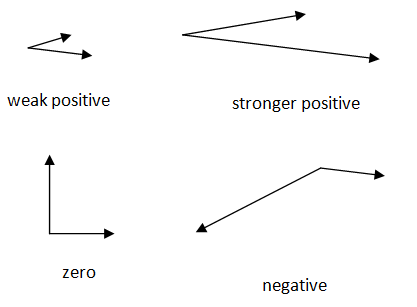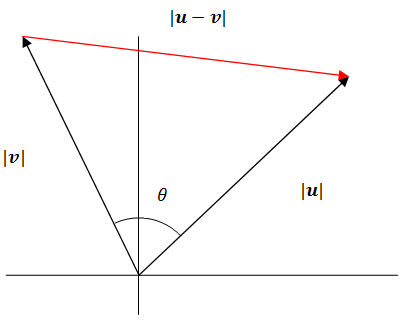# 7.4: Dot Product and Angle Between Two Vectors

•• Contributed by CK12
• CK12

While two vectors cannot be strictly multiplied like numbers can, there are two different ways to find the product between two vectors.  The cross product between two vectors results in a new vector perpendicular to the other two vectors.  You can study more about the cross product between two vectors when you take Linear Algebra.  The second type of product is the dot product between two vectors which results in a regular number.  Other names for the dot product include inner product and scalar product. This number represents how much of one vector goes in the direction of the other.  In one sense, it indicates how much the two vectors agree with each other.  This concept will focus on the dot product between two vectors.

What is the dot product between $$<-1,1>$$ and $$<4,4>?$$ What does the result mean?

### Properties of the Dot Product

The dot product is defined as:

$$u \cdot v=<u_{1}, u_{2}>\cdot<v_{1}, v_{2}>=u_{1} v_{1}+u_{2} v_{2}$$

This procedure states that you multiply the corresponding values and then sum the resulting products.  It can work with vectors that are more than two dimensions in the same way.

Before trying this procedure with specific numbers, look at the following pairs of vectors and relative estimates of their dot product.Notice how vectors going in generally the same direction have a positive dot product. Think of two forces acting on a single object. A positive dot product implies that these forces are working together at least a little bit. Another way of saying this is the angle between the vectors is less than $$90^{\circ}$$.

There are a many important properties related to the dot product. The two most important are 1) what happens when a vector has a dot product with itself and 2) what is the dot product of two vectors that are perpendicular to each other.

• $$v \cdot v=|v|^{2}$$
• $$v$$ and $$u$$ are perpendicular if and only if $$v \cdot u=0$$

The commutative property, $$u \cdot v=v \cdot u,$$ holds for the dot product between two vectors. The following proof is for two dimensional vectors although it holds for any dimensional vectors.

$$u=<u_{1}, u_{2}>$$
$$v=<v_{1}, v_{2}>$$

Then apply the definition of dot product and rearrange the terms.  The commutative property is already known for regular numbers so we can use that.

\begin{aligned} u \cdot v &=<u_{1}, u_{2}>\cdot\left\langle v_{1}, v_{2}\right\rangle \\ &=u_{1} v_{1}+u_{2} v_{2} \\ &=v_{1} u_{1}+v_{2} u_{2} \\ &=<v_{1}, v_{2}>\cdot<u_{1}, u_{2}>\\ &=v \cdot u \end{aligned}

The distributive property, $$u \cdot(v+w)=u v+u w,$$ also holds under the dot product. The following proof will work with two dimensional vectors although the property does hold in general.

$$u=<u_{1}, u_{2}>, v=<v_{1}, v_{2}>, w=<w_{1}, w_{2}>$$

\begin{aligned} u \cdot(v+w) &=u \cdot\left(<v_{1}, v_{2}>+<w_{1}, w_{2}>\right) \\ &\left.=u \cdot<v_{1}+w_{1}, v_{2}+w_{2}\right\rangle \\ &=<u_{1}, u_{2}>\cdot<v_{1}+w_{1}, v_{2}+w_{2}>\\ &=u_{1}\left(v_{1}+w_{1}\right)+u_{2}\left(v_{2}+w_{2}\right) \\ &=u_{1} v_{1}+u_{1} w_{1}+u_{2} v_{2}+u_{2} w_{2} \\ &=u_{1} v_{1}+u_{2} v_{2}+u_{1} w_{1}+u_{2} w_{2} \\ &=u \cdot v+v \cdot w \end{aligned}

The dot product can help you determine the angle between two vectors using the following formula.  Notice that in the numerator the dot product is required because each term is a vector.  In the denominator only regular multiplication is required because the magnitude of a vector is just a regular number indicating length.

$$\cos \theta=\frac{u \cdot v}{|u \| v|}$$

Watch the portion of this video focusing on the dot product:

### Examples

Example 1

Earlier, you were asked to find the dot product between the two vectors $$<-1,1>$$ and $$<4,4>$$. It can be computed as:

$$(-1)(4)+1(4)=-4+4=0$$

The result of zero makes sense because these two vectors are perpendicular to each other.

Example 2

Find the dot product between the following vectors: $$<3,1>\cdot<5,-4>$$

$$u=<3,5>$$

Example 3

Prove the angle between two vectors formula:

$$\cos \theta=\frac{u \cdot v}{|u||v|}$$\begin{aligned}|u-v|^{2} &=|v|^{2}+|u|^{2}-2|v||u| \cos \theta \\(u-v) \cdot(u-v) &=\\ u \cdot u-2 u \cdot v+v \cdot v &=\\|u|^{2}-2 u \cdot v+|v|^{2} &=\\-2 u \cdot v &=-2|v||u| \cos \theta \\ \frac{u \cdot v}{|u||v|} &=\cos \theta \end{aligned}

Example 4

Find the dot product between the following vectors.

$$(4 i-2 j) \cdot(3 i-8 j)$$

The standard unit vectors can be written as component vectors.

$$<4,-2>\cdot<3,-8>=12+(-2)(-8)=12+16=28$$

Example 5

What is the angle between $$u=<3,5>$$ and $$v=<2,8>?$$

Use the angle between two vectors formula.

$$u=<3,5>$$ and $$v=<2,8>$$

\begin{aligned} \frac{u \cdot v}{|u||v|} &=\cos \theta \\ \frac{<3,5>\cdot<2,8>}{\sqrt{34} \cdot \sqrt{68}} &=\cos \theta \\ \frac{6+40}{\sqrt{34} \cdot \sqrt{68}} &=\cos \theta \\ \cos ^{-1}\left(\frac{46}{\sqrt{34} \cdot \sqrt{68}}\right) &=\theta \\ 16.93^{\circ} & \approx \theta \end{aligned}

Review

Find the dot product for each of the following pairs of vectors.

1. $$<2,6>\cdot<-3,5>$$

2. $$<5,-1>\cdot<4,4>$$

3. $$<-3,-4>\cdot<2,2>$$

4. $$<3,1>\cdot<6,3>$$

5. $$<-1,4>\cdot<2,9>$$

Find the angle between each pair of vectors below.

6. $$<2,6>\cdot<-3,5>$$

7. $$<5,-1>\cdot<4,4>$$

8. $$<-3,-4>\cdot<2,2>$$

9. $$<3,1>\cdot<6,3>$$

10. $$<-1,4>\cdot<2,9>$$

11. What is $$v \cdot v ?$$

12. How can you use the dot product to find the magnitude of a vector?

13. What is $$0 \cdot v ?\ 14. Show that \((c u) \cdot v=u \cdot(c v)$$ where $$c$$ is a constant.

15. Show that $$<2,3>$$ is perpendicular to $$<1.5,-1>$$.